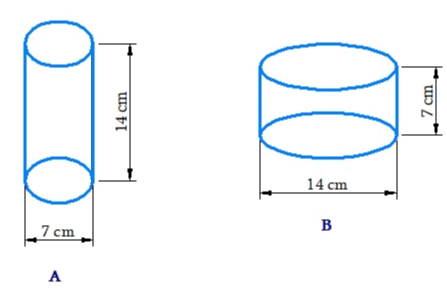# Ex.11.4 Q2 Mensuration Solution - NCERT Maths Class 8

Go back to  'Ex.11.4'

## Question

Diameter of cylinder $$A$$ is $$7 \rm \,cm,$$ and the height is $$14 \rm\,cm.$$ Diameter of cylinder $$B$$ is $$14 \rm\,cm$$ and height is $$7 \rm\,cm.$$ Without doing any calculations can you suggest whose volume is greater? Verify it by finding the volume of both the cylinders. Check whether the cylinder with greater volume also has greater surface area?Video Solution
Mensuration
Ex 11.4 | Question 2

## Text Solution

What is Known?

Dimensions of both cylinder $$A$$ and $$B$$ is given.

What is unknown?

Volume of both the cylinders.

Steps:

From the diagram, radius of cylinder $$A$$ is half of cylinder $$B.$$ so its volume will be lesser than that of cylinder B.

The curved surface area of both cylinders will be same, but the total surface area will be greater in the cylinder with greater radius.

\begin{align} A(r) = \frac{7}{2}\rm\,cm = 3.5\rm\,cm\end{align}

Height of cylinder $$A(h) = 14\rm\,\rm cm$$

Volume of cylinder $$A$$

\begin{align}&=\pi {r^2}h\\& = \frac{{22}}{7} \times 7 \times 7 \times 7\\&= 1078\,\,{\rm{c}}{{\rm{m}}^2}\end{align}

Curved surface area of cylinder $$A$$

\begin{align} &= 2\pi rh\\ &= 2 \times \frac{{22}}{7} \times 3.5 \times 14 = 308\,\,{\rm{c}}{{\rm{m}}^2}\end{align}

Total surface area of cylinder $$A$$

\begin{align}&= \! 2\pi r(r \! + \! h)\\&= \! 2\pi {r^2} \! + \! 2\pi rh\\&= \! \left[ {2 \! \times \! \frac{{22}}{7} \! \times \! 3.5 \! \times \! 3.5} \right]{\rm{c}}{{\rm{m}}^2} \! + \! 308{\rm{c}}{{\rm{m}}^2}\\ &= \! 385\,{\rm{c}}{{\rm{m}}^2}\end{align}

\begin{align}B(r) = \frac{{14}}{2}\,{\rm{cm}} = 7{\rm{cm}}\end{align}

Height of cylinder $${{B}}(h) = 7\rm\,cm$$

Volume of cylinder $$B$$

\begin{align} &=\pi {r^2}h\\ & = \frac{{22}}{7} \times 7 \times 7 \times 7\\ &= 1078\,\,{\rm{c}}{{\rm{m}}^2}\end{align}

Surface area of cylinder $$B$$

\begin {align} &= 2\pi rh\\ &= 2 \times \frac{{22}}{7} \times 7 \times 7\\& = 308\,\,\rm c{m^2}\end{align}

Total surface area of cylinder $$B$$

\begin{align} &= 2\pi r(r + h)\\& = 2\pi {r^2}h + 2\pi rh \\&= (2 \times \frac{{22}}{7} \times 7 \times 7)\,{\rm{c}}{{\rm{m}}^2} + 308\,{\rm{cm}}\\&=308\,{\rm{c}}{{\rm{m}}^2} + 308\,{\rm{c}}{{\rm{m}}^2} = 616\,{\rm{cm}}\end{align}

Thus, volume and surface area of cylinder $$B$$ is greater than cylinder $$A.$$

Learn from the best math teachers and top your exams

• Live one on one classroom and doubt clearing
• Practice worksheets in and after class for conceptual clarity
• Personalized curriculum to keep up with school